Linear Algebra MCQ Level - 2

# Linear Algebra MCQ Level - 2

Test Description

## 10 Questions MCQ Test Topic wise Tests for IIT JAM Physics | Linear Algebra MCQ Level - 2

Linear Algebra MCQ Level - 2 for IIT JAM 2023 is part of Topic wise Tests for IIT JAM Physics preparation. The Linear Algebra MCQ Level - 2 questions and answers have been prepared according to the IIT JAM exam syllabus.The Linear Algebra MCQ Level - 2 MCQs are made for IIT JAM 2023 Exam. Find important definitions, questions, notes, meanings, examples, exercises, MCQs and online tests for Linear Algebra MCQ Level - 2 below.
Solutions of Linear Algebra MCQ Level - 2 questions in English are available as part of our Topic wise Tests for IIT JAM Physics for IIT JAM & Linear Algebra MCQ Level - 2 solutions in Hindi for Topic wise Tests for IIT JAM Physics course. Download more important topics, notes, lectures and mock test series for IIT JAM Exam by signing up for free. Attempt Linear Algebra MCQ Level - 2 | 10 questions in 45 minutes | Mock test for IIT JAM preparation | Free important questions MCQ to study Topic wise Tests for IIT JAM Physics for IIT JAM Exam | Download free PDF with solutions
 1 Crore+ students have signed up on EduRev. Have you?
Linear Algebra MCQ Level - 2 - Question 1

### The system of the equations, x – 4y + 7z = 14 3x + 8y – 2z = 13 7x – 8y + 26z = 5 is :

Detailed Solution for Linear Algebra MCQ Level - 2 - Question 1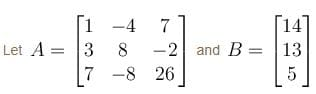Then, consider the augmented matrix,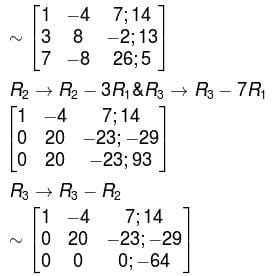∵  Rank A ≠ Rank [A : B]
∴  The system is inconsistent.

Linear Algebra MCQ Level - 2 - Question 2

### Consider the matrix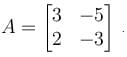Then

Detailed Solution for Linear Algebra MCQ Level - 2 - Question 2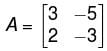tr(A) = 0, |A| = 1
∴ The characteristic polynomial will be,
t(t) = t2 + 1 = 0

Now, A is a matrix over the field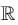.Then t(t) has no real roots. Thus A has no eigenvalues and no eigen vectors.

So, A is not diagonalisable overNow, if A is a matrix over C. Then,

t(t) = t2 – 1
has two roots i and i

Thus, A  has two distinct eigenvalues i and i.

Hence A has two independent eigenvectors. Accordingly, A is diagonalisable over  C.

The correct answer is: A is diagonalisable over C but notLinear Algebra MCQ Level - 2 - Question 3

### For what values of  k, the given system of equations will have a unique solution? 2x + 3ky + (3k + 4)z = 0 x + (k + 4)y + (4k + 2)z = 0 x + 2(k + 1)y + (3k + 4)z = 0

Detailed Solution for Linear Algebra MCQ Level - 2 - Question 3

For the unique solution. |A| should be non-zero where A  is the coefficient matrix. i.e.,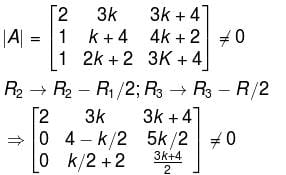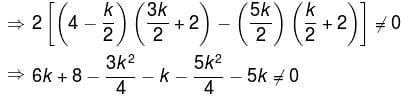⇒ 2k2 + 8 ≠ 0
⇒ k2 ≠ 4
⇒  k ≠ ± 2

The correct answer is: k ≠ ±2

Linear Algebra MCQ Level - 2 - Question 4

Which of the following will satisfy the given system?
2x1 – 3x2 – 6x3 – 5x4 + 2x5 = 7
x3 + 3x4 – 7x5 = 6
x4 – 2x5 = 1

Detailed Solution for Linear Algebra MCQ Level - 2 - Question 4

Consider the augmented matrix,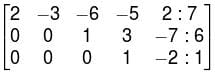We can see that the pivot elements exists in columns 1, 3 and 4

So, x2 and x5  are free variables.

Given, 2x1 – 3x2 – 6x3 – 5x4 + 2x5 = 7     ...(1)
x3 + 3x4 – 7x5 = 6     ...(2)
x4 – 2x5 = 1       ...(3)
from (3),
x4 = 1 + 2x5
from (2),
x3 + 3(1 + 2x5) – 7x5 = 6
x3 = 3 + x5

from (1),
2x1 – 3x2 –6(3 + x5) –5(1 + 2x5) + 2x5 = 7
⇒  2x1 – 3x2 – 18 – 6x5 – 5 - 10x5 + 2x5 = 7
⇒ 2x1 – 3x2 – 23 – 14x5 = 7
⇒ 2x1 = 30 + 3x2 + 14x5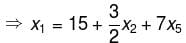∵  x2  and x5 are free variables, Let  x2 = x5 = 0
then  (15, 0, 3, 1, 0)T  becomes one of the solutions.
The correct answer is: (15, 0, 3, 1, 0)T

Linear Algebra MCQ Level - 2 - Question 5

The system of equations,
x + y + z = 0
2x – y – 3z = 0
3x – 5y + 4z = 0
x + 17y + 4z = 0

Will have :

Detailed Solution for Linear Algebra MCQ Level - 2 - Question 5

Consider the coefficient matrix,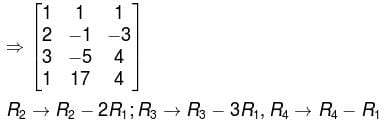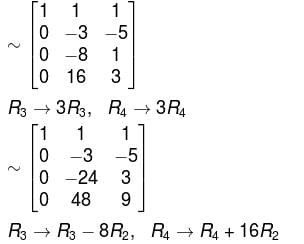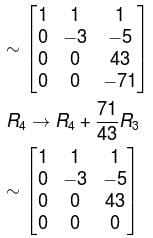Rank of the coefficient matrix is 3 which is equal to the number of unknowns.

The given system of equations possesses non zero solution.

The correct answer is: Unique solution

Linear Algebra MCQ Level - 2 - Question 6

Choose the correct option about the system,
3x + y + z = 8
x + y – 2z = –5
x + y + z = 6
–2x + 2y – 3z = –7

Detailed Solution for Linear Algebra MCQ Level - 2 - Question 6

Consider the augmented matrix,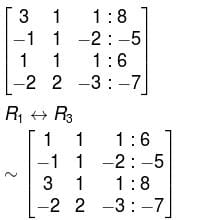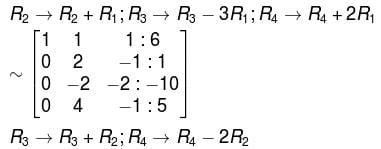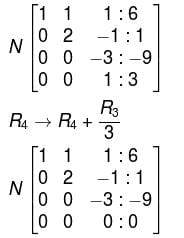∴  Rank of coefficient matrix = Rank of augmented matrix =  Number of unknowns
Hence,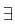unique solution, the system reduces to,
–3z = – 9  ⇒  z = 3
2y – z = 1  ⇒  y = 2
x + y + z = 6  ⇒  x = 1
∴  The solution is  (1, 2, 3)T.

The correct answer is: The system is consistent having unique solution,  (1, 2, 3)T

Linear Algebra MCQ Level - 2 - Question 7

A necessary and sufficient condition that values, not all zero may be assigned to n variables x1, x2, ......., xn  so that the homogeneous equations, ai1x1 + ai2x2 + ..... + ainxn = 0  (i = 1, 2,.....n) hold simultaneously, is :

Detailed Solution for Linear Algebra MCQ Level - 2 - Question 7

For the scalars,  ai1, ai2, ..........., ain  (i,= 1, 2,.....,n)
not all zero such that  ai1x1 + ai2x2 + ...... + ain xn = 0
⇔ that  {x1, x2,..........,xn}  is linearly dependent.
⇒ Rank of coefficient matrix will be less than. Hence, minor of order n in the matrix or determinant of the coefficient matrix will be zero.

The correct answer is: |aij|n × n = 0

Linear Algebra MCQ Level - 2 - Question 8

Consider the following statements about the given system of equations,
x + 2y + 3z  = 0
3x + 4y + 4z  = 0
7x + 10y + 12z  = 0

and choose the correct one

Detailed Solution for Linear Algebra MCQ Level - 2 - Question 8

Coefficient matrix to the given system of equation is,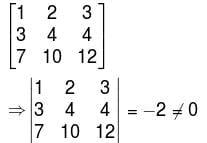Hence, the rank of the coefficient matrix is 3 which is equal to the number of unknown variables in the system.
∴  The system has a unique solution.

The correct answer is: The given system is consistent and has a unique solution.

Linear Algebra MCQ Level - 2 - Question 9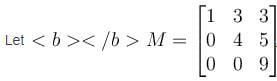Then

Detailed Solution for Linear Algebra MCQ Level - 2 - Question 9

Since, M  is upper triangular, M2 will also be upper triangular and hence, both of them will be diagonalisable.

The correct answer is: Both M and M2  are diagonalisable

Linear Algebra MCQ Level - 2 - Question 10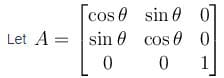Then which of the following option is correct.

Detailed Solution for Linear Algebra MCQ Level - 2 - Question 10

Determinant  A = cos2 θ +sin2 θ = 1
Hence, A is non-singular and A-1exists

The correct answer is: A is non singular and A–1 exists

## Topic wise Tests for IIT JAM Physics

217 tests
Information about Linear Algebra MCQ Level - 2 Page
In this test you can find the Exam questions for Linear Algebra MCQ Level - 2 solved & explained in the simplest way possible. Besides giving Questions and answers for Linear Algebra MCQ Level - 2, EduRev gives you an ample number of Online tests for practice

## Topic wise Tests for IIT JAM Physics

217 tests(Scan QR code)